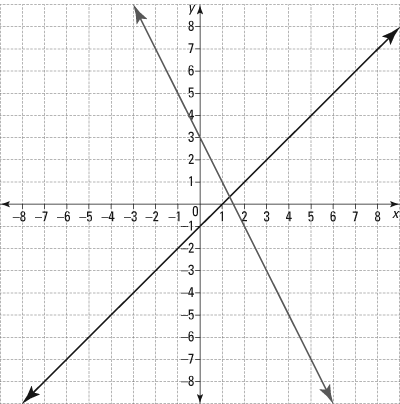##### TASC For DummiesIf a question from the TASC Math exam asks you to solve for the solution to a system of equations, one useful approach is to graph the system of equations.

Graphically, the solution is the point or points where the lines or curves intersect. This means to solve a system of equations (linear, quadratic, and so on) by graphing, you follow these steps:

1. Graph each function independently but on the same coordinate plane.
2. Look for the point or points where the functions intersect.
3. Test the points you identified by substituting them into all original equations. While this step is optional, it's highly recommended because graphs can be drawn inaccurately if generated by hand.

## Practice question

1. Which system of equations is represented by the following graph?A. y = 2x – 1; y = x + 3 B. y = –2x + 3; y = x – 1 C. y = –x + 3; y = 2x – 1 D. y = –x –1; y = 2x + 3